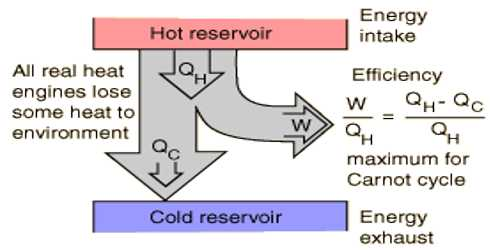Physics

# Efficiency of EngineEfficiency of Engine

Before discussing the efficiency of an engine we need to know what are heat engine and its working principle. The machine by which heat energy is transformed into mechanical energy is called heat engine. For example, vapor engine, petrol engine, diesel engine etc. How much an engine is workable is known for its efficiency. The efficiency of an engine is measured by the amount of absorbed heat and heat energy transformed into work.

That means the ratio of the heat energy transformed into work by the engine and the amount of absorbed heat energy by the engine is called the efficiency of the engine.

i.e., Efficiency of the engine, η =

[Transformed heat energy into work by the engine /Absorbed heat energy by the engine]

= (Q1 – Q2) / Q1

Calculation of efficiency: Suppose, working substances in the heat engine absorb Q1 heat from the source at temperature T1 and work done by it is W and the rest amount of heat Q2 is rejected in the heat sink at temperature T2. Then the amount of heat transformed into work = Q1 – Q2.

Thermal efficiency of the engine, η =

[Heat converted into work in the engine / Absorbed heat energy by the engine]

= (Q1 – Q2) / Q1 = 1 – (Q2 / Q1) … …. (1)

From the equation (1) it is seen that as less the value of Q2 is the more is the value of η.

Normally the efficiency of an engine is expressed in terms of percentage.

Thermal efficiency of the engine, η = [1 – (Q2 / Q1)] x 100%

The heat engine can never convert heat into work or mechanical energy. Normally, the efficiency of a heat engine is 30%. For an ideal engine, it can be shown.

η = (Q1 – Q2) / Q1 = (T1 – T2) / T1; here working substance receives heat at temperature T1K and rejects heat at temperature T2K.

Explanation: The efficiency of an engine can never be 100%.

The equation for the efficiency cat a heat engine or Carnot’s engine.

η = (T1 – T2) / T1 x 100%

From this equation, it is seen that the efficiency of the engine depends on the temperatures of heat source T1 and heat sine T2. It does not depend on the working substance. Again efficiency of all the reversible engines working between two fixed temperatures is same. In that equation, T1 > (T1 – T2), so the efficiency of an engine can never be 100%. If the difference between the temperatures of the heat source and heat sink increases, efficiency also increases.